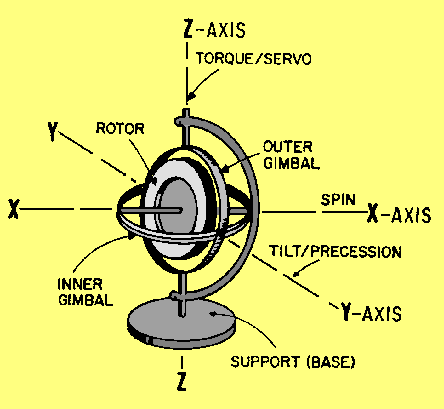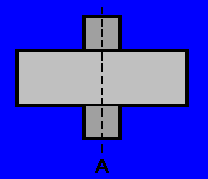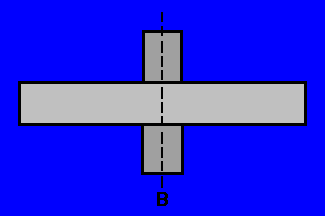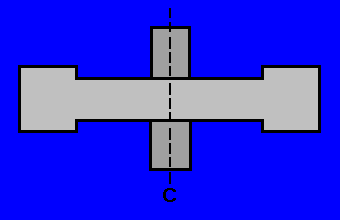Basic Gyro ElementsCustom SearchBASIC GYRO ELEMENTS The gyro shown in figure 3-5 is a basic, universally mounted gyro, sometimes called a free gyro. Its components are rotor, inner gimbal, outer gimbal, and base or support. Gimbals are devices that permit the rotor to assume any position and retain that position when the support is tipped or repositioned. Note that in figure 3-5, the support may be moved about all axes without the rotor position being disturbed. Figure 3-5. - Basic universally mounted gyro.As you know, gravity is a force that acts along parallel lines upon each particle of matter. A plot of the resultant gravitational force on a body such as a gyro would be equivalent to the sum of these separate forces. The point at which the resultant force is applied is called the CENTER OF GRAVITY. To have a balanced gyro, the center of gravity must be located at the intersection of the three axes of the gyro. RIGIDITY A gyroscope is a spinning body that tends to keep its spin axis rigidly pointed in a fixed direction in space. What do we mean by "fixed direction in space"? A fixed direction on Earth is by no means fixed in space, because the Earth turns once on its axis every 24 hours, and makes a complete revolution around the sun every year. The sun itself is moving through space, taking the Earth and the other planets with it. Because of these motions, the expression "fixed direction in space" as used in this explanation is theoretical. For all practical purposes, we can say a line from the Earth to a distant star is a fixed direction in space. If the spin axis of a spinning gyro is pointed at a distant star, it will remain pointed at the star as the Earth turns. Gyro rigidity is the strength with which a gyro resists any external force that would tilt its rotor spin axis. There are three factors that determine gyro rigidity: weight of the rotor, distribution of this weight, and rotor speed. The gyro can be considered as an enclosed mechanical system. The energy in the system is equal to the input energy. Hence the energy necessary to spin the gyro rotor is contained in the rotor as angular momentum, which is a function of rotor weight and the speed of rotor rotation. The heavier the gyro rotor, the larger the torque necessary to spin it, and the greater the angular momentum of the rotor. If we have two rotors with identical shapes but different weights spinning at the same velocity, the heavier of the two will be more rigid in its spin axis since it has the greater angular momentum. Now let's look at the effect of weight distribution in the rotor of a gyro. Consider three rotors of the same weight, as shown in figure 3-6, view (A), view (B), and view (C), with the diameter of one rotor half the diameter of the other two. Now, when we spin these rotors at the same speed, we find that the rotors with the greater diameter are much more rigid than the one with the smaller diameter. Next, we find that we can make the rotors equally rigid by causing the rotor with the smaller diameter to spin faster than the larger rotors. Thus rigidity depends both on speed and distribution of weight. The weight of the larger rotor being farther away from the axis of spin causes it to be more rigid. This effect is even more pronounced if we shape the rotor as shown in view C of figure 3-6. Shifting as much weight as possible to the outer rim of the rotor increases rigidity even further. Figure 3-6A. - Gyro rotors with equal weight and unequal diameters.Figure 3-6B. - Gyro rotors with equal weight and unequal diameters.Figure 3-6C. - Gyro rotors with equal weight and unequal diameters.Q.6 A universally mounted gyro has how many gimbals?Q.7 What factors determine the rigidity of a gyro?Q.8 Which gyro rotor in figure 3-6, view (A) view (B) view (C), will have the greatest rigidity if all are rotated at the same speed?Integrated Publishing, Inc. - A (SDVOSB) Service Disabled Veteran Owned Small Business